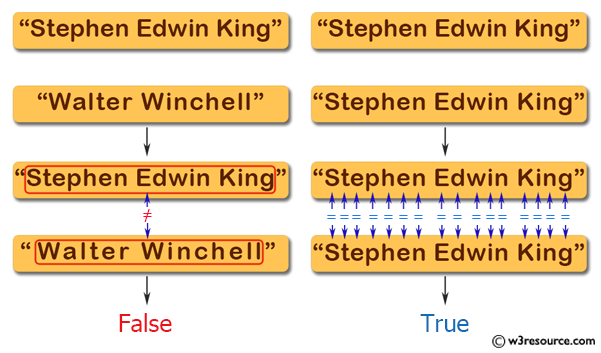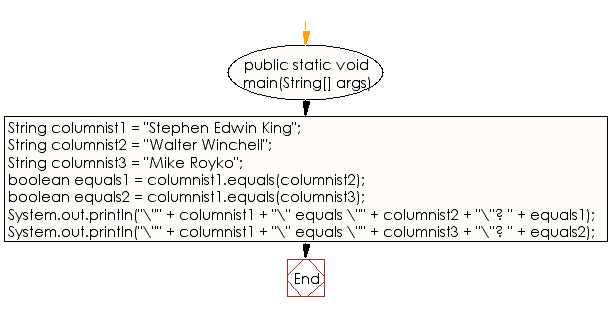﻿ Java exercises: Check whether two String objects contain the same data - w3resource# Java String Exercises: Check whether two String objects contain the same data

## Java String: Exercise-13 with Solution

Write a Java program to check whether two String objects contain the same data.

Pictorial Presentation:Sample Solution:

Java Code:

``````public class Exercise13 {

public static void main(String[] args)
{
String columnist1 = "Stephen Edwin King";
String columnist2 = "Walter Winchell";
String columnist3 = "Mike Royko";

// Are any of the above Strings equal to one another?
boolean equals1 = columnist1.equals(columnist2);
boolean equals2 = columnist1.equals(columnist3);

// Display the results of the equality checks.
System.out.println("\"" + columnist1 + "\" equals \"" +
columnist2 + "\"? " + equals1);
System.out.println("\"" + columnist1 + "\" equals \"" +
columnist3 + "\"? " + equals2);
}
}
```
```

Sample Output:

```"Stephen Edwin King" equals "Walter Winchell"? false
"Stephen Edwin King" equals "Mike Royko"? false
```

Flowchart:Visualize Java code execution (Python Tutor):

Java Code Editor:

Improve this sample solution and post your code through Disqus

What is the difficulty level of this exercise?

﻿

## Java: Tips of the Day

Count the number of occurrences of a char in a String:

```int count = StringUtils.countMatches("a.b.c.d", ".");
```

Ref: https://bit.ly/2OTy6sG